Home Practice
For learners and parents For teachers and schools
Textbooks
Full catalogue
Pricing SupportLog in

We think you are located in United States. Is this correct?

# 4.6 Addition, elimination and substitution reactions

## 4.6 Addition, elimination and substitution reactions (ESCKY)

Mechanisms and arrow-pushing are not required by CAPS. The learners need to know the types of reactants, the types of reactions and the reaction conditions.

We will study three main types of reactions - addition, elimination and substitution.

• An addition reaction occurs when two or more reactants combine to form a single product. This product will contain all the atoms that were present in the reactants. Addition reactions occur with unsaturated compounds.

The general equation for an addition reaction: $$\text{A}+\text{B}$$ $$\to$$ $$\text{C}$$

Notice that C is the final product with no A or B remaining as a residue.

• An elimination reaction occurs when a reactant is broken up into two products. Elimination reactions occur with saturated compounds.

The general equation for an elimination reaction: $$\text{A}$$ $$\to$$ $$\text{B}+\text{C}$$

• A substitution reaction occurs when an exchange of elements in the reactants takes place. The initial reactants are transformed or swopped around to give a final product.

The general equation for a substitution reaction: $$\text{AB} + \text{CD}$$ $$\to$$ $$\text{AD} + \text{BC}$$

Some specific examples of these reactions are given in the following pages.

Remember from the section on saturated and unsaturated structures that you can perform tests to determine if a compound is saturated or not. If bromine water or potassium permanganate are decolourised by a compound the compound is unsaturated, if they are not decolourised the compound is saturated.

#### Hydrohalogenation

Hydrohalogenation involves the addition of a hydrogen atom and a halogen atom to an unsaturated compound (containing a carbon-carbon double bond). An example is given in Figure 4.76. X can be fluorine (F), chlorine (Cl), bromine (Br) or iodine (I).

If more than one product is possible the major product will be the compound where:

Remember that a $$\color{red}{\textbf{tertiary carbon atom}}$$ is bonded to three other carbon atoms, a $$\color{orange}{\textbf{secondary carbon atom}}$$ is bonded to two other carbon atoms, and a $$\color{blue}{\textbf{primary carbon atom}}$$ is bonded to one other carbon atom. So tertiary is the most substituted, secondary is less substituted than tertiary and more substituted than primary, and primary is the least substituted.

• the $$\color{darkgreen}{\textbf{hydrogen atom}}$$ is added to $$\color{blue}{\textbf{least substituted carbon atom}}$$

• i.e. the carbon atom with the least number of carbon atoms bonded to it

• the $$\color{purple}{\textbf{halogen atom}}$$ is added to the $$\color{red}{\textbf{more substituted carbon atom}}$$

• i.e. the carbon atom with the most number of carbon atoms bonded to it

This is shown in Figure 4.77.

Major and minor products

The major product of a reaction is the product that is most likely to form. Minor products are those that are less likely to form.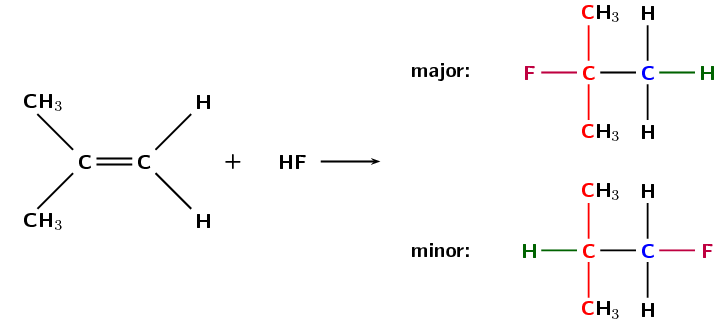Figure 4.77: The hydrohalogenation of 2-methylpropene to form 2-fluoro-2-methylpropane (major product) and 1-fluoro-2-methylpropane (minor product).

Reaction conditions:

• no water present in the reaction

#### Halogenation

Halogenation is very similar to hydrohalogenation but a diatomic halogen molecule is added across the double bond. An example is given in Figure 4.78.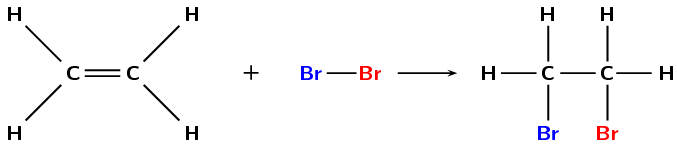Figure 4.78: The reaction between ethene and bromine to form 1,2-dibromoethane.

#### Hydration

A hydration reaction involves the addition of water ($$\text{H}_{2}\text{O}$$) to an unsaturated compound. This is one way of preparing an alcohol from the corresponding alkene (Figure 4.79).

If more than one product is possible the major product will be the compound where:

• the $$\color{darkgreen}{\textbf{hydrogen atom}}$$ is added to the $$\color{blue}{\textbf{least substituted carbon atom}}$$

• the $$\color{purple}{\textbf{hydroxyl anion}}$$ ($$\text{OH}^{-}$$) is added to the $$\color{red}{\textbf{more substituted carbon atom}}$$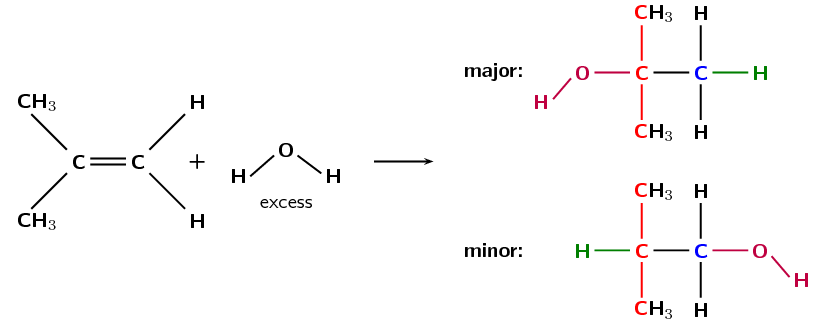Figure 4.80: The hydration of 2-methylpropene to form 2-methylpropan-2-one (major product) and 2-methyl-propan-1-one (minor product).

Reaction conditions:

• water must be present in excess

• an acid catalyst is needed for this reaction to take place

• the catalyst that is most commonly used is phosphoric acid ($$\text{H}_{3}\text{PO}_{4}$$)

Fermentation can refer to the conversion of sugar to alcohol using yeast (a fungus). The process of fermentation produces items such as wine, beer and yogurt. To make wine, grape juice is fermented to produce alcohol. This reaction is shown below:

$$\text{C}_{6}\text{H}_{12}\text{O}_{6}$$ $$\to$$ $$2\text{CO}_{2} + 2\text{C}_{2}\text{H}_{5}\text{OH}+$$ energy

The learners should focus their research on the chemistry behind these fermentation processes. They can either present their findings as a discussion to the class, or in a short (1-page) report format. The research should be on local-level fermentation processes, not industrial scale breweries.

## Formation of alcohol through fermentation

Ethanol can also be formed through the process of fermentation. In almost every country there is a local product that people make by fermenting local fruits. For example in Malawi people ferment potatoes, sugar cane or maize to form katchasu. Here in South Africa people ferment marula fruit to make mampoer, grapes to make witblits and sorghum to make beer.

• Research the chemistry behind the fermentation process.

• What other plants do people ferment?

• Fermentation leads to acid formation, research why this occurs.

• Sorghum is also used to make sour porridge. How is it made?

#### Hydrogenation

Hydrogenation involves adding hydrogen $$(\text{H}_{2})$$ to an alkene. During hydrogenation the double bond is broken (as with hydrohalogenation and halogenation) and more hydrogen atoms are added to the molecule. A specific example is shown in Figure 4.81.

Reaction conditions:

• a catalyst such as platinum ($$\text{Pt}$$), palladium ($$\text{Pd}$$) or nickel ($$\text{Ni}$$) is needed for these reactions

• heating is required

• the reaction must be done under an inert atmosphere, not air (e.g. $$\text{N}_{2}(\text{g})$$ atmosphere)

The hydrogenation of vegetable oils to form margarine is another example of this addition reaction (see Figure 4.82).

#### Polymerisation reactions

A polymer is made up of lots of smaller units called monomers. When these monomers are added together, they form a polymer. One way for polymerisation to occur is through an addition reaction. More details are given later in this chapter in Plastics and polymers (Section 4.7).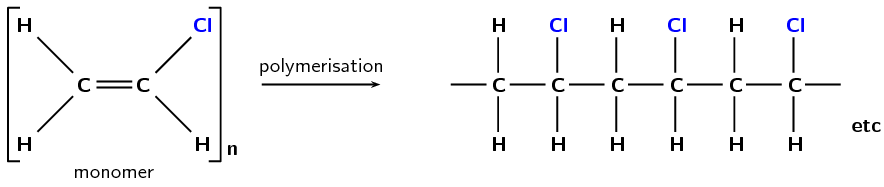Figure 4.83: The polymerisation of vinyl chloride monomers to form a polyvinyl chloride polymer.

Polyvinyl chloride (Figure 4.83) is used in construction and in clothing, as well as having many other uses.

Textbook Exercise 4.26

The following diagram shows the reactants in an addition reaction.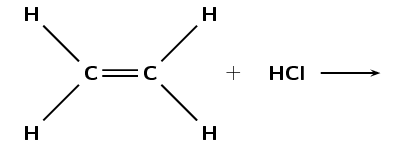Draw the structural representation of the final product in this reaction.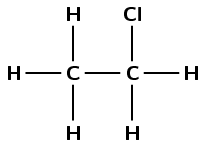What is the condensed structural representation of the product?

$$\text{CH}_{3}\text{CH}_{2}(\text{Cl})$$

The following diagram shows the reactants in an addition reaction.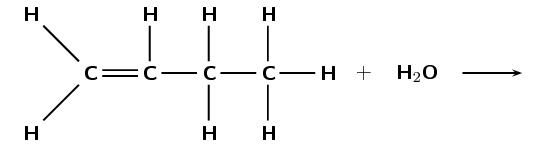Draw the structural representation of the major product in this reaction and give reasons.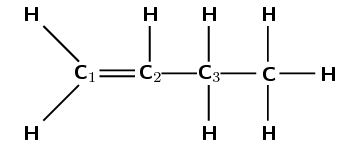The hydroxyl anion ($$\text{OH}^{-}$$) can bond to carbon one, or carbon two. Carbon atom one is bonded only to carbon atom two. Carbon atom two is bonded to carbon atom one and carbon atom three.

Therefore, carbon atom one is less substituted and carbon atom two is more substituted. In the major product, the hydroxyl anion will add to the more substituted carbon atom (two).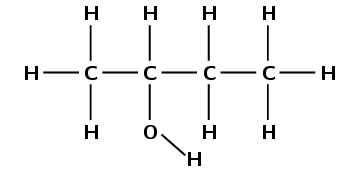Draw the structural representation of the minor product in this reaction and give reasons.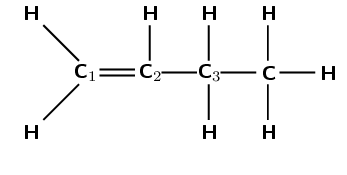The hydroxyl anion ($$\text{OH}^{-}$$) can bond to carbon one, or carbon two. Carbon atom one is bonded only to carbon atom two. Carbon atom two is bonded to carbon atom one and carbon atom three.

Therefore, carbon atom one is less substituted and carbon atom two is more substituted. In the minor product, the hydroxyl anion will add to the less substituted carbon atom (one).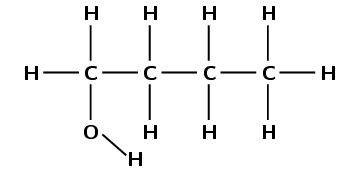What type of addition reaction is this reaction?

Hydration reaction

Which types of homologous series can undergo addition reactions?

Any series where the functional group contains double or triple bonds (e.g. alkenes, alkynes).

Is this a saturated or unstaturated series?

Unsaturated

### Elimination reactions (ESCM2)

1. Dehydrohalogenation

Reflux is a technique whereby a reaction solution is placed in a container with a small opening at the top. A tube that is constantly being cooled is connected to the opening. The solution is heated to approximately its boiling point, but any gases produced are condensed in the tube and fall back into the container. This way the reaction rate can be increased without the loss of material.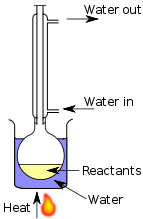In dehydrohalogenation a haloalkane is exposed to a base, the base then helps the elimination of the halogen and a hydrogen atom. A double bond is formed (alkane $$\to$$ alkene). Dehydrohalogenation is considered the opposite of hydrohalogenation. The elimination of iodine from iodoethane is an example of dehydrohalogenation (Figure 4.84).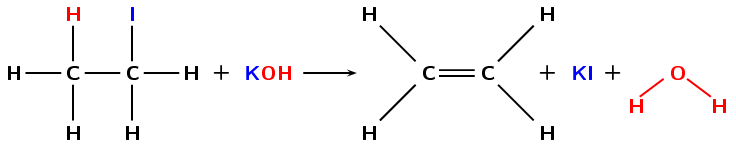Figure 4.84: The dehydrohalogenation of iodoethane with potassium hydroxide ($$\text{KOH}$$) to form ethene, potassium iodide ($$\text{KI}$$) and water.

See hydrolysis in the substitution reactions section for more information on what happens when different reaction conditions are used.

In order for elimination to occur the following reaction conditions must be used:

• heat under reflux (approximately $$\text{70}$$$$\text{°C}$$)
• a concentrated, strong base (e.g. $$\text{NaOH}$$, $$\text{KOH}$$)
• the base must be dissolved in pure ethanol (hot ethanolic base)

Dihalogenated saturated compounds can also undergo elimination reactions to become unsaturated, losing one halogen and one hydrogen atom. In the example below (Figure 4.85), an atom of hydrogen and chlorine are eliminated from the original compound to form an unsaturated haloalkene and hydrochloric acid.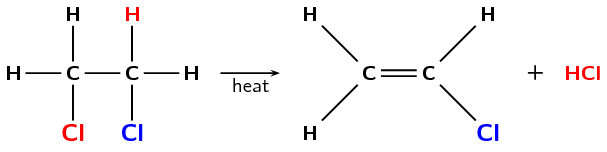Figure 4.85: The elimination of hydrochloric acid ($$\text{HCl}$$) from 1,2-dichloroethane to form chloroethene.

If more than one product is possible the major product will be the compound where:

• the hydrogen atom is removed from the $$\color{red}{\textbf{more substituted carbon atom}}$$
• i.e. the carbon that is bonded to the most number of carbon atoms

See Figure 4.86.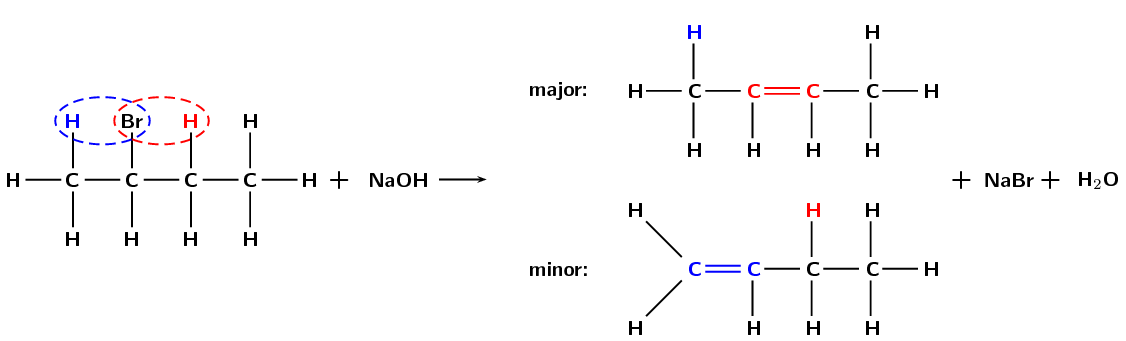Figure 4.86: The dehydrohalogenation of 2-bromobutane to form but-2-ene (major product) and but-1-ene (minor product).
2. Dehydration of an alcohol

Sulfuric acid ($$\text{H}_{2}\text{SO}_{4}$$) is a dehydrating agent and is often used to prepare dried fruits.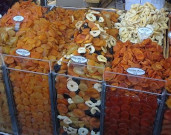During the dehydration of an alcohol the hydroxyl ($$-\text{OH}$$) group and a hydrogen atom are eliminated from the reactant. A molecule of water is formed as a product in the reaction, along with an alkene (Figure 4.87). This can be thought of as the reverse of a hydration (addition) reaction.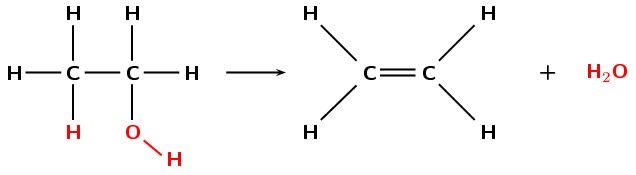Figure 4.87: The dehydration of ethanol to form ethene and $$\text{H}_{2}\text{O}$$.

Reaction conditions:

• an excess of a strong acid catalyst (generally $$\text{H}_{2}\text{SO}_{4}$$ or $$\text{H}_{3}\text{PO}_{4}$$)
• high temperature (approximately $$\text{180}$$$$\text{°C}$$)

If more than one elimination product is possible the major product will be the compound where:

• the hydrogen atom is removed from the carbon atom bonded to the most number of carbon atoms (i.e., the $$\color{blue}{\textbf{more substituted carbon atom}}$$)

This is shown in Figure 4.88.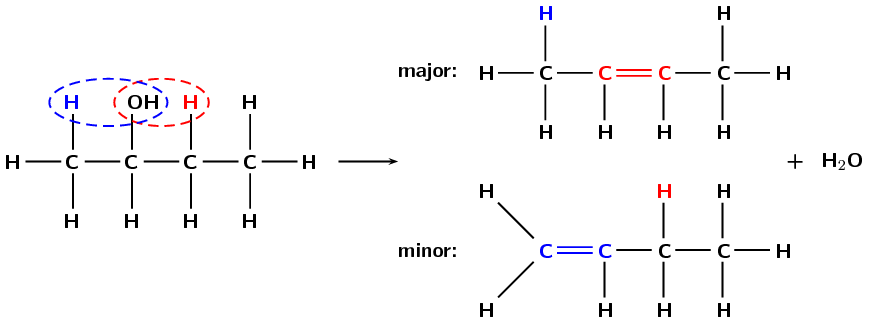Figure 4.88: The dehydration of butan-2-ol to form but-2-ene (major product) and but-1-ene (minor product).

## Elimination reactions

Textbook Exercise 4.27

What two compounds do you need for a dehydrohalogenation reaction?

Haloalkane and a base

What carbon atom will the hydrogen atom be removed from in a dehydration reaction (major product)?

The more substituted carbon atom of those atoms directly bonded to the carbon atom the hydroxyl group is attached to. That is the carbon atom bonded to the most other carbon atoms.

Are the reactants in an elimination reaction saturated or unsaturated?

saturated

Name the elimination reaction that is considered the reverse of a hydration addition reaction

dehydration reaction (of an alcohol)

### Substitution reactions (ESCM3)

Some simple examples of substitution reactions are shown below:

$$\text{CH}_{4} + \text{Cl}_{2}$$ $$\to$$ $$\text{CH}_{3}\text{Cl} + \text{HCl}$$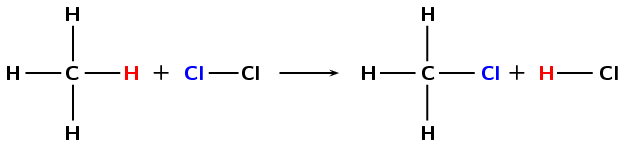A chlorine atom (from $$\text{Cl}_{2}$$) and a hydrogen atom (from $$\text{CH}_{4}$$) are exchanged to create new products ($$\text{CH}_{3}\text{Cl}$$ and $$\text{HCl}$$).
1. Formation of haloalkanes

Haloalkanes can be formed when the hydroxyl ($$-\text{OH}$$) group of an alcohol is replaced by a halogen atom (X = Cl, Br). This reaction works best with tertiary alcohols where it can occur at room temperature (Figure 4.89)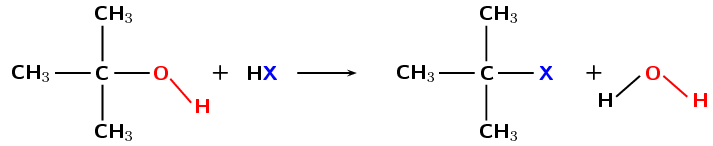Figure 4.89: The formation of a tertiary haloalkane from a tertiary alcohol by a substitution with HX (X = Cl, Br).

A tertiary carbon atom is attached to three other carbon atoms. A secondary carbon atom is attached to two other carbon atoms while a primary carbon atom is attached to one other carbon atom. In Figure 4.89 the carbon is attached to the functional group as well as three other carbon atoms. This makes it a tertiary alcohol on the left of the arrow and a tertiary haloalkane on the right.

It is possible for a secondary or primary alcohol to form a secondary or primary haloalkane as well (see Figure 4.90) but this requires high temperatures and the reaction is very slow.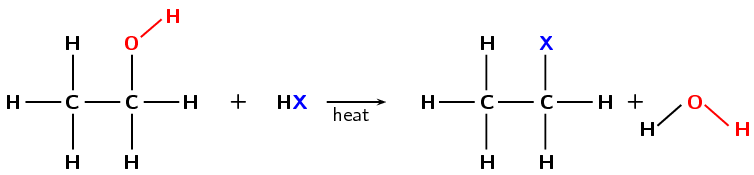Figure 4.90: The formation of a primary haloethane from ethanol by a substitution with $$\text{HX}$$ (X = Cl, Br).
2. Hydrolysis

In a previous section we saw the hydration of an ethene to form an alcohol. That is an addition reaction. Alcohols can also be formed through a substitution reaction with a haloalkane. In the example given in Figure 4.91 the haloalkane would be dissolved in water.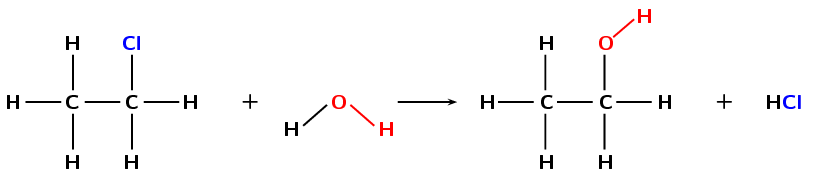Figure 4.91: The formation of ethanol from chloroethane through a substitution reaction with water.

See dehydrohalogenation in the elimination reactions section for more information on what happens when different reaction conditions are used.

Using a base (e.g. $$\text{KOH}$$, $$\text{NaOH}$$) dissolved in water and warming the solution would increase the rate of the reaction (Figure 4.92). However, in order for substitution to occur the following reaction conditions must be used:

• low temperatures (around room temperature)

• a dilute solution of a strong base (e.g. $$\text{NaOH}$$)

• the solution must be aqueous (in water)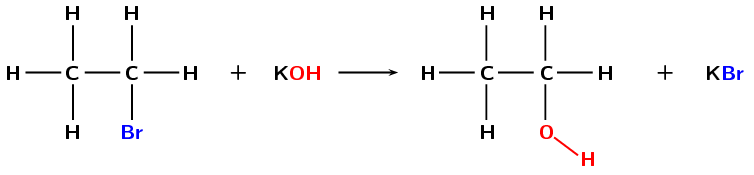Figure 4.92: The formation of ethanol from bromoethane through a substitution reaction with potassium hydroxide ($$\text{KOH}$$).

If the concentration of the base is too high then the halogen atom and a hydrogen atom could be eliminated (dehydrohalogenation).

3. Formation of haloalkanes from alkanes

Another way of forming a haloalkane involves the removal of a hydrogen atom from a saturated compound. The hydrogen atom is replaced by a halogen ($$\text{F}$$, $$\text{Cl}$$, $$\text{Br}$$ or $$\text{I}$$) to form a haloalkane (Figure 4.93). As alkanes are not very reactive light is needed for this reaction to take place.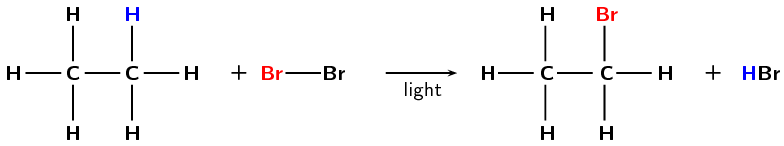Figure 4.93: The formation of bromoethane from ethane through a substitution reaction with $$\text{Br}_{2}$$.

Refer to the section on saturated and unsaturated structures for more information on why alkanes are not very reactive.

Reaction conditions:

• energy in the form of light

## Substitution reactions

Textbook Exercise 4.28

When forming haloalkanes through a substitution reaction with alcohols is it best to use a tertiary, secondary or primary alcohol? Give a reason for your answer.

A tertiary alcohol. The reaction works best with tertiary alcohols (it can occur at room temperature). Higher temperatures are required for secondary and primary alcohols and the reaction is much slower.

What is a substitution reaction?

An exchange of elements in the reactants must take place. The initial reactants are transformed or 'swopped around' to give the final products.

Write the general equation for a substitution reaction using the reactants $$\text{WX}$$ and $$\text{YZ}$$

$$\text{WX} + \text{YZ}$$ $$\to$$ $$\text{WZ} + \text{XY}$$ or $$\text{WX} + \text{YZ}$$ $$\to$$ $$\text{WY} + \text{XZ}$$

## Addition, elimination and substitution reactions

Textbook Exercise 4.29

What type of reaction is a hydration reaction?

What is the most common catalyst used in this reaction?

$$\text{H}_{3}\text{PO}_{4}$$

Name one of the most common catalysts used in a hydrogenation reaction.

Are alkanes reactive compared to alkenes and alkynes?

No

In a haloalkane reaction an alkane and a halogen react. What condition is necessary for this reaction to take place?

Energy in the form of light

Look at the general equations given below and answer the questions that follow:

haloalkane + base $$\to$$ A + B

Name A and B for a hydrolysis reaction.

alcohol and salt (containing the halogen atom).

What type of reaction is a hydrolysis reaction?

substitution

haloalkane + base $$\to$$ A + B + C

Name A, B and C for a dehydrohalogenation reaction.

alkene, salt and water

What type of reaction is a dehydrohalogenation reaction?

elimination

Fill in the reaction conditions required for dehydrohalogenation and hydrolysis reactions in the table below:

 Dehydrohalogenation Hydrolysis Temperature Solvent Concentration of base
 Dehydrohalogenation Hydrolysis Temperature high ($$\text{70}$$$$\text{°C}$$) low (room temperature) Solvent alcoholic aqueous Concentration of base concentrated dilute

Refer to the diagram below and then answer the questions that follow: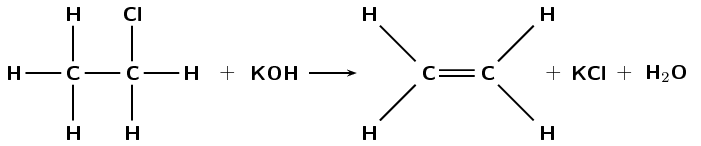What type of reaction is this reaction?

Elimination

A single bond becomes a double bond and a hydrogen atom and a chlorine atom are eliminated from the reactant.

What are the required reaction conditions?

Heat, under reflux (approximately $$\text{70}$$$$\text{°C}$$), a concentrated alcoholic (ethanol) solution of a strong base (e.g. $$\text{NaOH}$$ or $$\text{KOH}$$).

Write the equation for the following reactions using the condensed structural representation. Say what type of reaction they are:

The dehydration of hexan-2-ol ($$\text{CH}_{3}\text{CH}(\text{OH})\text{CH}_{2}\text{CH}_{2}\text{CH}_{2}\text{CH}_{3}$$)

$$\text{CH}_{3}\text{CH}(\text{OH})\text{CH}_{2}\text{CH}_{2}\text{CH}_{2}\text{CH}_{3}$$ $$\to$$ $$\text{CH}_{3}\text{CHCHCH}_{2}\text{CH}_{2}\text{CH}_{3} + \text{H}_{2}\text{O}$$

hexan-2-ol $$\to$$ hex-2-ene + water (major product)

OR

$$\text{CH}_{3}\text{CH}(\text{OH})\text{CH}_{2}\text{CH}_{2}\text{CH}_{2}\text{CH}_{3}$$ $$\to$$ $$\text{CH}_{2}\text{CHCH}_{2}\text{CH}_{2}\text{CH}_{2}\text{CH}_{3} + \text{H}_{2}\text{O}$$

hexan-2-ol $$\to$$ hex-1-ene + water (minor product)

Elimination

The hydration of pent-1-ene ($$\text{CH}_{2}\text{CHCH}_{2}\text{CH}_{2}\text{CH}_{3}$$)

$$\text{CH}_{2}\text{CHCH}_{2}\text{CH}_{2}\text{CH}_{3} + \text{H}_{2}\text{O}$$ $$\to$$ $$\text{CH}_{3}\text{CH}(\text{OH})\text{CH}_{2}\text{CH}_{2}\text{CH}_{3}$$

pent-1-ene + water $$\to$$ pentan-2-ol (major product)

OR

$$\text{CH}_{2}\text{CHCH}_{2}\text{CH}_{2}\text{CH}_{3} + \text{H}_{2}\text{O}$$ $$\to$$ $$\text{CH}_{2}(\text{OH})\text{CH}_{2}\text{CH}_{2}\text{CH}_{2}\text{CH}_{3}$$

pent-1-ene + water $$\to$$ pentan-1-ol (minor product)

The hydrolysis of 2-fluorobutane ($$\text{CH}_{3}\text{CH}(\text{F})\text{CH}_{2}\text{CH}_{3}$$) with water (no base).

$$\text{CH}_{3}\text{CH}(\text{F})\text{CH}_{2}\text{CH}_{3} + \text{H}_{2}\text{O}$$ $$\to$$ $$\text{CH}_{3}\text{CH}(\text{OH})\text{CH}_{2}\text{CH}_{3} + \text{HF}$$

2-fluorobutane + water $$\to$$ butan-2-ol + hydrofluoric acid

Substitution

The following reaction takes place: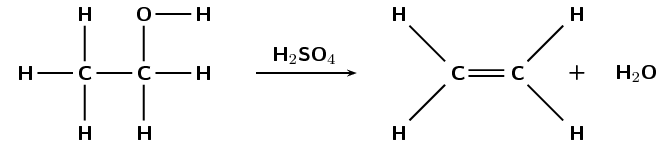What type of reaction is this?

Elimination

A saturated compound (alcohol) becomes an unsaturated compound (alkene) with a molecule of water eliminated from the reactant (alcohol).

Write the equations for the following reactions using condensed structural representations. State the type of reaction as well.

The hydrogenation of propene ($$\text{CH}_{2}\text{CHCH}_{3}$$)

$$\text{CH}_{2}\text{CHCH}_{3} + \text{H}_{2}$$ $$\to$$ $$\text{CH}_{3}\text{CH}_{2}\text{CH}_{3}$$

propene + hydrogen $$\to$$ propane

The formation of a haloalkane from:

butan-1-ol ($$\text{CH}_{2}(\text{OH})\text{CH}_{2}\text{CH}_{2}\text{CH}_{3}$$) and hydrochloric acid ($$\text{HCl}$$)

$$\text{CH}_{2}(\text{OH})\text{CH}_{2}\text{CH}_{2}\text{CH}_{3} + \text{HCl}$$ $$\to$$ $$\text{CH}_{2}(\text{Cl})\text{CH}_{2}\text{CH}_{2}\text{CH}_{3} + \text{H}_{2}\text{O}$$

butan-1-ol + hydrochloric acid $$\to$$ 1-chlorobutane + water

Substitution

2-methylpent-2-ene ($$\text{CH}_{3}\text{C}(\text{CH}_{3})\text{CHCH}_{2}\text{CH}_{3}$$) and hydroiodic acid ($$\text{HI}$$). Indicate the major and minor products and give reasons.

In the major product the hydrogen will add to the less substituted carbon atom (third carbon atom), and the iodine will add to the more substituted carbon atom (second carbon atom):

$$\text{CH}_{3}\text{C}(\text{CH}_{3})\text{CHCH}_{2}\text{CH}_{3} + \text{HI}$$ $$\to$$ $$\text{CH}_{3}\text{C}(\text{CH}_{3})(\text{I})\text{CH}_{2}\text{CH}_{2}\text{CH}_{3}$$

2-methylpent-2-ene + hydroiodic acid $$\to$$ 2-iodo-2-methylpentane

In the minor product the hydrogen will add to the more substituted carbon atom (second carbon atom), and the iodine will add to the less substituted carbon atom (third carbon atom):

$$\text{CH}_{3}\text{C}(\text{CH}_{3})\text{CHCH}_{2}\text{CH}_{3} + \text{HI}$$ $$\to$$ $$\text{CH}_{3}\text{CH}(\text{CH}_{3})\text{CH}(\text{I})\text{CH}_{2}\text{CH}_{3}$$

2-methylpent-2-ene + hydroiodic acid $$\to$$ 3-iodo-2-methylpentane

Write balanced equations for the following reactions involving hept-3-ene ($$\text{CH}_{3}\text{CH}_{2}\text{CHCHCH}_{2}\text{CH}_{2}\text{CH}_{3}$$) and name the product.

Hydrohalogenation, choose any halogen

$$\text{CH}_{3}\text{CH}_{2}\text{CHCHCH}_{2}\text{CH}_{2}\text{CH}_{3} + \text{HX}$$ $$\to$$ $$\text{CH}_{3}\text{CH}_{2}\text{CH}(\text{X})\text{CH}_{2}\text{CH}_{2}\text{CH}_{2}\text{CH}_{3}$$

hept-3-ene + hydrochloric acid (e.g.) $$\to$$ 3-chloroheptane

OR

$$\text{CH}_{3}\text{CH}_{2}\text{CHCHCH}_{2}\text{CH}_{2}\text{CH}_{3} + \text{HX}$$ $$\to$$ $$\text{CH}_{3}\text{CH}_{2}\text{CH}_{2}\text{CH}(\text{X})\text{CH}_{2}\text{CH}_{2}\text{CH}_{3}$$

hept-3-ene + hydrochloric acid (e.g.) $$\to$$ 4-chloroheptane

Either product is possible as the carbons on either side of the double bond are equally substituted (bonded to two carbon atoms and one hydrogen atom).

The dehydrohalogenation with potassium hydroxide ($$\text{KOH}$$) of the product from c) i

$$\text{CH}_{3}\text{CH}_{2}\text{CH}(\text{X})\text{CH}_{2}\text{CH}_{2}\text{CH}_{2}\text{CH}_{3} + \text{KOH}$$ $$\to$$

$$\text{CH}_{3}\text{CHCHCH}_{2}\text{CH}_{2}\text{CH}_{2}\text{CH}_{3}$$

3-chloroheptane + potassium hydroxide $$\to$$ hept-2-ene

OR

$$\text{CH}_{3}\text{CH}_{2}\text{CH}(\text{X})\text{CH}_{2}\text{CH}_{2}\text{CH}_{2}\text{CH}_{3} + \text{KOH}$$ $$\to$$

$$\text{CH}_{3}\text{CH}_{2}\text{CHCHCH}_{2}\text{CH}_{2}\text{CH}_{3}$$

3-chloroheptane + potassium hydroxide $$\to$$ hept-3-ene

Either product is possible as the carbons on either side of the double bond are equally substituted (bonded to two carbon atoms and one hydrogen atom).

OR

$$\text{CH}_{3}\text{CH}_{2}\text{CH}_{2}\text{CH}(\text{X})\text{CH}_{2}\text{CH}_{2}\text{CH}_{3} + \text{KOH}$$ $$\to$$

$$\text{CH}_{3}\text{CH}_{2}\text{CHCHCH}_{2}\text{CH}_{2}\text{CH}_{3}$$

4-chloroheptane + potassium hydroxide $$\to$$ hept-3-ene

Elimination

Halogenation, choose any halogen

$$\text{CH}_{3}\text{CH}_{2}\text{CHCHCH}_{2}\text{CH}_{2}\text{CH}_{3} + \text{X}_{2}$$ $$\to$$

$$\text{CH}_{3}\text{CH}_{2}\text{CH}(\text{X})\text{CH}(\text{X})\text{CH}_{2}\text{CH}_{2}\text{CH}_{3}$$

hept-3-ene + bromine (e.g.) $$\to$$ 3,4-dibromoheptane

The dehydrohalogenation of the product from c) iii

$$\text{CH}_{3}\text{CH}_{2}\text{CH}(\text{X})\text{CH}(\text{X})\text{CH}_{2}\text{CH}_{2}\text{CH}_{3}$$ $$\to$$

$$\text{CH}_{3}\text{CH}_{2}\text{C}(\text{X})\text{CHCH}_{2}\text{CH}_{2}\text{CH}_{3} + \text{HX}$$

e.g. 3,4-dibromoheptane $$\to$$ 3-bromohept-3-ene + hydrobromic acid

OR

$$\text{CH}_{3}\text{CH}_{2}\text{CH}(\text{X})\text{CH}(\text{X})\text{CH}_{2}\text{CH}_{2}\text{CH}_{3}$$ $$\to$$

$$\text{CH}_{3}\text{CH}_{2}\text{CHC}(\text{X})\text{CH}_{2}\text{CH}_{2}\text{CH}_{3} + \text{HX}$$

e.g. 3,4-dibromoheptane $$\to$$ 4-bromo-hept-3-ene + hydrobromic acid

Elimination